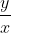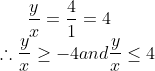# Numbers and Algebra - Numbers and Algebra Section 1

>>>>>>>>>>Numbers and Algebra Section 1

• A

0• B

1• C

2• D

x2 - y2• Option : B
• Explanation : x- y3- 3xy = x3 - y3 - 3xy(x y)....as (x - y) = 1 = (x - y)3= 1.• Option : D
• Explanation :

y 4 3 2 1 -1 -3 -4

x 6 5 4 3 1 -1 -2

Hence, minimum value ofAnd maximum value of• A

h = a!• B

h = n!• C

h ≥ n!• D

h ≥ a * n• Option : D
• Explanation : (a. n)! = product of n consecutive natural numbers starting from 'a' which is atleast divisible by n!. (n)! = product of n consecutive natural numbers. For n = 2 : (a. n)! = a(a + 1) and n! = 2 a(a + 1) is divisible by 2!. For n = 3 : (a n)! = a(a + 1)(a + 2) and n! = 6. One of the factors of a(a + 1)(a + 2) is divisible by 3 and other by 2. Thus, proceeding in this manner, (a. n)! and n! have HCF = n! ∴ H = n!.

I. a2 has three positive integer factors.

II. ab has four positive integer factors.

III. a3 has four positive integer factors.

• A

I and II only• B

II and III only• C

All of these• D

None of these• Option : C
• Explanation :

Factors of a2 are 1. a and a2.
Factors of ab are 1, a, b and ab.
Factors of a3 are 1. a. a2 and a3.

• A

a + b + c• B

4b2• C

abc• D

a+ b2• Option : B
• Explanation :

x+ y2 + z2-2xy - 2xz + 2yz
= (x - y - z)2
=(b+c-c+a-a+b)2= 4b2